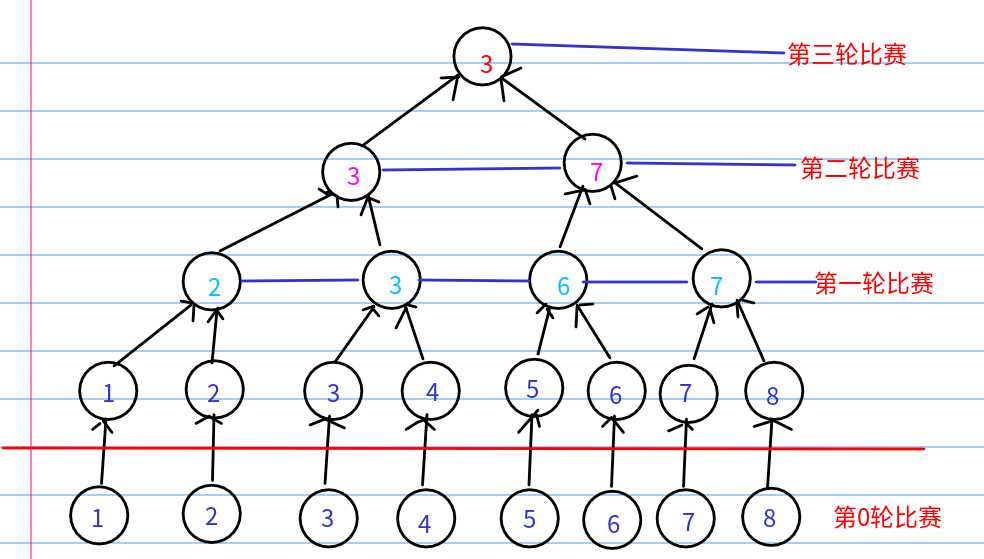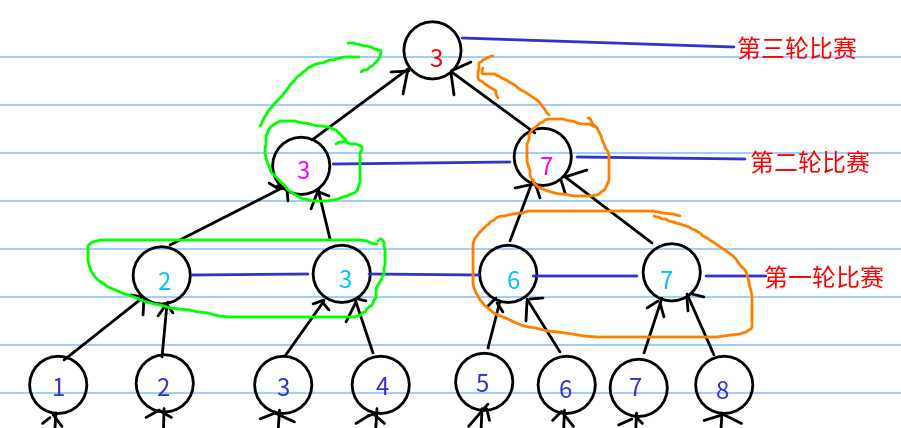# POJ3071(Football)--概率DP

en....网上当然也后不少解题报告，但是很多直接给出状态转移方程和贴出代码，而少了其中重要的推断过程，我觉得不是很好。所以自己给写一个较为详细的过程DP的含义确定了，那么，状态转移方程怎么来呢？？DP[i][j] =  DP[i-1][j] * DP[i-1][k] * ???;

DP[I-1][j]：表示本轮的赢家j在上一轮获胜的概率，上一轮一定是获胜了的再能到这一轮

DP[i-1][k]：表示本轮赢家j遇见的对手k，k在上一轮获胜的概率，k也晋级到了这一轮

???：这个表示什么呢???因为此时DP[i][j]表示这一轮是j获胜的概率，所以j战胜了k，那么胜率是多少呢??

`1 dp[i][j] += dp[i-1][j] * dp[i-1][k] * G[j][k];`

3 /(1<<3) == 7/(1<<3)  ==>0     &&    3 /(1<<2 ) != 7/(1<<2)

`((j / (1<<i)) == (k / (1<<i)) ) && ( j /(1<<(i-1))  != (k / (1<<(i-1))) ) ```` 1 #include<iostream>
2 #include<cstdio>
3 #include<algorithm>
4 #include<cstring>
5 using namespace std;
6 const int maxn = 130;
7 int n;//总过(1<<n)个队伍
8 double G[maxn][maxn];//存放各个队伍间的胜率关系 G[i][j]即队伍i击败队伍j的概率
9 double dp[maxn][maxn];//dp[i][j]表示在第i轮第j只球队获胜的概率
10
11 void DP()
12 {
13     for(int i = 0;i < (1<<n);i++)//算出每个队伍第0场的胜率，都为1.0
14     {
15         dp[i] = 1.0;
16     }
17     for(int i = 1;i <= n;i++)//决出冠军最多的轮数，一棵二叉树的深度-1
18     {
19         for(int j = 0; j < (1<<n);j++)//遍历每个队伍，计算出当前轮次的胜率
20         {
21             dp[i][j]= 0.0;//注意别忘了这个init
22             for(int k = 0; k < (1<<n);k++)//遍历可能交手的队伍
23             {
24                 if(j == k)
25                 {
26                     continue;//自己与自己不交手
27                 }
28                 //关键部分，j和k在决赛轮相遇，而在决赛轮之前它们是不会遇见的，即j和k是来自两个不同的小组的队伍
29                 if(((j / (1<<i)) == (k / (1<<i)) ) && ( j /(1<<(i-1))  != (k / (1<<(i-1))) ) )
30                 {
31                     dp[i][j] += dp[i-1][j] * dp[i-1][k] * G[j][k];
32                     // j上一轮获胜的概率  *  k上一轮获胜的概率 * 最后一轮j战胜k的概率
33                 }
34             }
35         }
36     }
37 }
38
39 int main()
40 {
41     while((scanf("%d",&n)&& n != -1))
42     {
43         for(int i =0 ;i< (1<<n);i++)
44         {
45             for(int j = 0;j < (1<<n);j++)
46             {
47                 scanf("%lf",&G[i][j]);
48             }
49         }
50         DP();
51         int ans = 0;//保存胜率最大的队伍编号
52         for(int j = 1 ;j < (1<<n);j++)//遍历每一只队伍
53         {
54             if(dp[n][ans] < dp[n][j])//更新胜率大的队伍
55             {
56                 ans = j;
57             }
58         }
59         cout<<ans+1<<endl;
60     }
61     return 0;
62 }```
View Code

POJ3071(Football)--概率DP

(0)
(0)